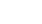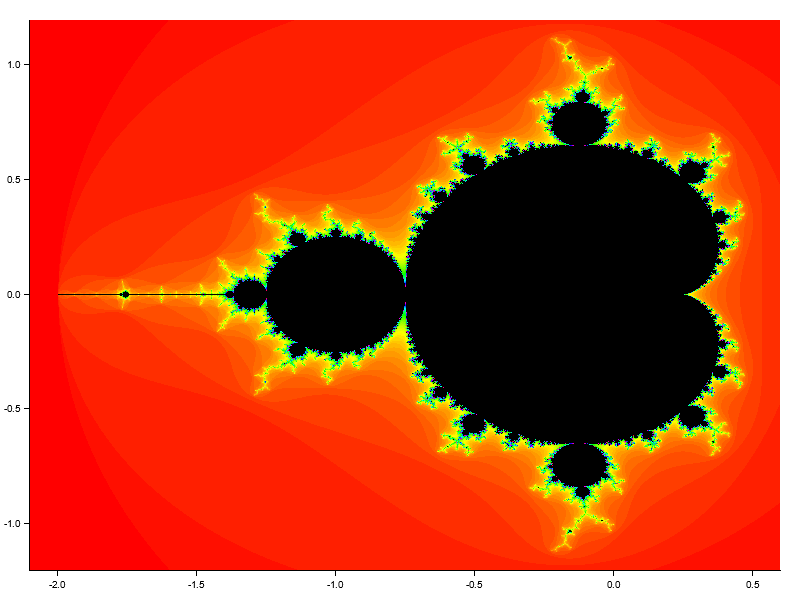# Shaking off the Rust### March 6th, 2022Difficulty: Beginner

Shaking off the Rust is a series of exercises with the Rust programing language. The purpose of the series is to improve both my and my dear reader’s abilities with Rust by building things. Plus, by actually building stuff, we'll learn about an array of technological concepts in the process. In this installment, we’re going to create our own Mandelbrot set.

This installment’s Github repo: https://github.com/josht-jpg/rust_mandelbrot_set

### What is a Mandelbrot Set?

The Mandelbrot set is a mathematical object discovered by Benoit Mandelbrot in 1980 .

The Mandelbrot set lives in the domain of complex numbers. Last week’s installment of SOTR was all about complex numbers. This installment will build on last week’s. If you haven’t gone through it already, you may want to give it a read, or just grab the code from Github:

To understand what the Mandelbrot set actually is, first consider the function $f_c(z) = z^2 + c$﻿, where $z$﻿ and $c$﻿ are complex numbers.

Let $c = -1$﻿, and consider $f(0)$﻿. We have that $f(0) = 0^2 + (-1) = -1$﻿. Now plug the result, $-1$﻿, back into $f$﻿:

$f_{-1}(-1) = (-1)^2 + (-1) = 0$﻿.

So when $c = -1$﻿ and we start with $f(0)$﻿, we can keep plugging the previous result of the function back into itself, and we will not encounter any numbers with a large magnitude. But if we do the same with $c=1$﻿, the results will become increasingly larger:

$f_1(0) = (0)^2 + 1 = 1. \hspace{3mm} f_1(1) = (1)^2 + 1 = 2. \hspace{3mm} f_1(2) = (2)^2 + 1 = 5...$﻿

So, depending on $c$﻿, one of two things will happen when we begin with $f(0)$﻿ and repeatedly plug the previous result of the function back into itself:

1. The results of $f$﻿ under iteration will eventually have a magnitude larger than 2.
1. The results of $f$﻿ under iteration will always have a magnitude less than or equal to 2.

The Mandelbrot set is the set of all complex numbers where case 2 holds .

If you’d like an explanation of the Mandelbrot set on video, this one from Holly Krieger on Numberphile is the best you’ll get:

### Getting Started

We’ll start by creating a new library in Cargo:

cargo new mandelbrot --lib
cd mandelbrot

There are two dependencies we’ll need.

The first is our suite of functions on complex numbers from last week’s installment of SOTR. Make sure the complex_numbers library from last week is in the same directory as your mandelbrot library.

The second dependency is the plotters library, which is a lovely library for rendering figures, plots, and charts. We’ll use it to visualize the Mandelbrot set.

So add those dependencies to your Cargo.toml file:

//  Cargo.toml

/*...*/

[dependencies]
complex_numbers={path="../complex_numbers"}
plotters="^0.3.1"

And in our lib.rs, we’ll bring in everything we need from those dependencies:

//  lib.rs

use plotters::prelude::*;

### Coding the Mandelbrot Set

We’ll start by creating a function called mandelbrot, which will calculate the number of iterations before the magnitude of $f_z$﻿ becomes larger than $2$﻿ (if it does become larger than $2$﻿).

mandelbrot takes in

• z - a complex number,

and

• num_iterations - the number of iterations before z is determined to be a member of the Mandelbrot set.

So if mandelbrot(z, num_iterations) == num_iterations, then we classify z as being a member of the Mandelbrot set.

I’ll begin with pseudocode for mandelbrot, followed by a Rust implementation.

mandelbrot arguments {
z: a complex number,
num_iterations: the number of iterations before z is determined to be a member of the Mandelbrot set
}

function mandelbrot(z, num_iterations)
returning the number of iterations it takes z to diverge (or num_iterations, if z does not diverge)
{
diverge_count = 0

z1 = z
while diverge_count <= num_iterations {
if magnitude(z1) > 2 {
return diverge_count
}

z1 = z1^2 + z
diverge_count = diverge_count + 1
}
return num_iterations
}


And here’s some Rust for ya:

//  lib.rs

/*...*/

fn mandelbrot(z: &Complex, num_iterations: u32) -> u32 {
let mut diverge_count: u32 = 0;

let mut z1 = Complex(0., 0.);
while diverge_count <= num_iterations {
if magnitude(&z1) > 2. {
return diverge_count;
}

diverge_count += 1;
}
num_iterations
}

I’ll note a few things about this code. If you have an intermediate or better understanding of Rust, these bullets won’t contain anything new for you (and you won’t be any worse off by skipping them).

• The first argument, z: &Complex is a reference to a complex number (using the Complex type we defined in the complex_numbers library). In Rust, a reference is a memory address that will lead us to a value of a particular type. And because Rust is incredible, that value is guaranteed to be valid . References are created by the ampersand operator &. References are an integral part of Rust. If you are unfamiliar with them, I recommend reading chapter 4.2 of The Rust Programming Language.
• The second argument, num_iterations: u32, is a 32-bit unsigned integer. Meaning, it can be any integer from $0$﻿ to $2^{32} - 1$﻿ $(2^{32} - 1 = 4,294,967,295)$﻿. If we wanted a 32-bit interger that could be negative, we’d use a signed integer type: i32.
• We declare the diverge_count variable with let mut. In Rust, variables are immutable by default. That is, if we declare a value like this: let foo = some_expression, we will not be able to change foo. Declaring variables with let mut allows us to change them.

Before we begin bragging that we’ve created our very own Mandelbrot set with Rust, let’s run some tests to make sure we got it right:

//  lib.rs

/*...*/

#[cfg(test)]
mod tests {
use super::*;

#[test]
fn mandelbrot_test() {
const NUM_ITERATIONS: u32 = 20;

//  Not in the mandelbrot set
let z1 = Complex(0.25, 0.75);
assert_ne!(mandelbrot(&z1, NUM_ITERATIONS), NUM_ITERATIONS);

let z2 = Complex(-1., 0.5);
assert_ne!(mandelbrot(&z2, NUM_ITERATIONS), NUM_ITERATIONS);

//  In the mandelbrot set
let z3 = Complex(0., 0.);
assert_eq!(mandelbrot(&z3, NUM_ITERATIONS), NUM_ITERATIONS);

let z4 = Complex(1. / 8., -1. / 8.);
assert_eq!(mandelbrot(&z4, NUM_ITERATIONS), NUM_ITERATIONS);
}

}

If running cargo test succeeds, you are free to start bragging.

### Plotting our Mandelbrot Set

The fine people who created the Plotters library have provided an example of how the Mandelbrot set looks with the plotters library. I’ve adapted the code to work with our mandelbrot function:

//  lib.rs

/*...*/

const OUT_FILE_NAME: &'static str = "mandelbrot.png";
fn draw_mandelbrot() -> Result<(), Box<dyn std::error::Error>> {
let root = BitMapBackend::new(OUT_FILE_NAME, (800, 600)).into_drawing_area();

root.fill(&WHITE)?;

let mut chart = ChartBuilder::on(&root)
.margin(20 as i32)
.x_label_area_size(10 as i32)
.y_label_area_size(10 as i32)
.build_cartesian_2d(-2.1f64..0.6f64, -1.2f64..1.2f64)?;

chart
.configure_mesh()
.disable_x_mesh()
.disable_y_mesh()
.draw()?;

let plotting_area = chart.plotting_area();

let range = plotting_area.get_pixel_range();

let samples = (range.0.end - range.0.start, range.1.end - range.1.start);
let (real, complex) = (chart.x_range(), chart.y_range());

let step = (
(real.end - real.start) / samples.0 as f64,
(complex.end - complex.start) / samples.1 as f64,
);

const NUM_CONVERGE: u32 = 100;

for k in 0..(samples.0 * samples.1) {
let z = Complex(
real.start + step.0 * (k % samples.0) as f64,
complex.start + step.1 * (k / samples.0) as f64,
);

let count = mandelbrot(&z, NUM_CONVERGE);

let Complex(a, b) = z;

if count != NUM_CONVERGE {
plotting_area.draw_pixel((a, b), &HSLColor(count as f64 / 100.0, 1.0, 0.5))?;
} else {
plotting_area.draw_pixel((a, b), &BLACK)?;
}
}

root.present().expect("Unable to write result to file, please make sure 'plotters-doc-data' dir exists under current dir");
println!("Result has been saved to {}", OUT_FILE_NAME);

Ok(())
}

To plot your own Mandelbrot set, put this test function in lib.rs and run cargo test:

#[cfg(test)]
mod tests {

/*...*/

#[test]
fn draw_mandelbrot_test() {
draw_mandelbrot().unwrap()
}
}

You should see a file called mandelbrot.png in your /mandelbrot directory, and it should look lovely.Feel free to email me if you have any issues: joshtaylor361@gmail.com.

I’m hoping to create an installment of SOTR where we use Rust and WebAssembly to build an applet in which users can interact with the Mandelbrot set.

Thanks again for coding with me. Like any other skill, it takes countless practice sessions to become a great programmer. I hope you found this one enjoyable.

## Support Me

Creating and running Shaking off the Rust is one of the most fulfilling things I do. But it's exhausting. By supporting me, even if it's just a dollar, you'll allow me to put more time into building this series. I really appreciate any support.

The only way to support me right now is by sponsoring me on Github. I'll probably also set up Patreon and Donorbox pages soon.

Thank you so much!